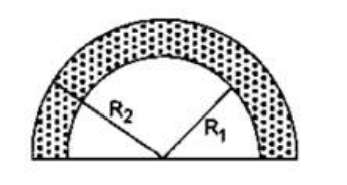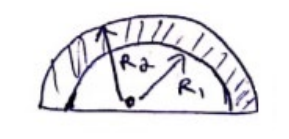# Solve the following :

Question:

Find the center of mass of a uniform plate having semicircular inner and outer boundaries of radii $R_{1}$ and $R_{2}$.Solution:C.O.M of shaded region $=\frac{\int r \cdot d m_{R_{2}}-\int r . d m_{R_{1}}}{M_{R_{2}}-M_{R_{1}}}$

$M_{R_{\Omega}}=\int d m R_{2}=\rho\left(\frac{4 \pi R_{2}^{2}}{2}\right) l$

$M_{R_{1}}=\int d m R_{1}=\rho\left(\frac{4 \pi R_{1}^{2}}{2}\right) l$

${ }_{\{} P=$ density of material $\}$

$\{\mid=$ thickness of disks $\}$

$d m_{R_{2}}=\rho l d A$

$=\rho \cdot l \cdot r \sin \theta d r d \theta\left\{r=0\right.$ to $\left.R_{2}\right\}$

$d m_{R_{1}}=\rho \cdot l \cdot r \sin \theta d r d \theta\left\{r=0\right.$ to $\left.R_{1}\right\}$

\begin{aligned} & \frac{\rho l\left[\int_{0}^{\pi_{2}} \int_{0}^{\pi} x^{2} \sin \theta d r d \theta-\int_{0}^{\pi_{1}} \int_{0}^{\pi} r^{2} \sin \theta d r d \theta\right]}{\rho_{2}^{\frac{[\pi}{2}}\left(R_{2}^{2}-R_{1}^{2}\right)} \\=& \end{aligned}

$=\frac{2}{\pi} \times \frac{2}{3} \frac{\left(R_{2}^{3}-R_{1}^{3}\right)}{\left(R_{2}^{2}-R_{1}^{3}\right)}$

$=\frac{4}{=3 \pi} \frac{\left(R_{2}-R_{1}\right)^{3}+3 R_{1} R_{2}\left(R_{2}-R_{1}\right)}{\left(R_{1}+R_{2}\right)\left(R_{2}-R_{1}\right)}$

$=\frac{4}{3 \pi} \frac{R_{2}^{2}+R_{2} R_{1}+R_{1}^{2}}{R_{1}+R_{2}}$

above center